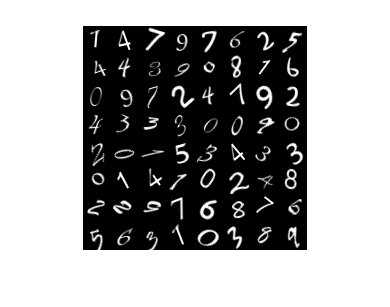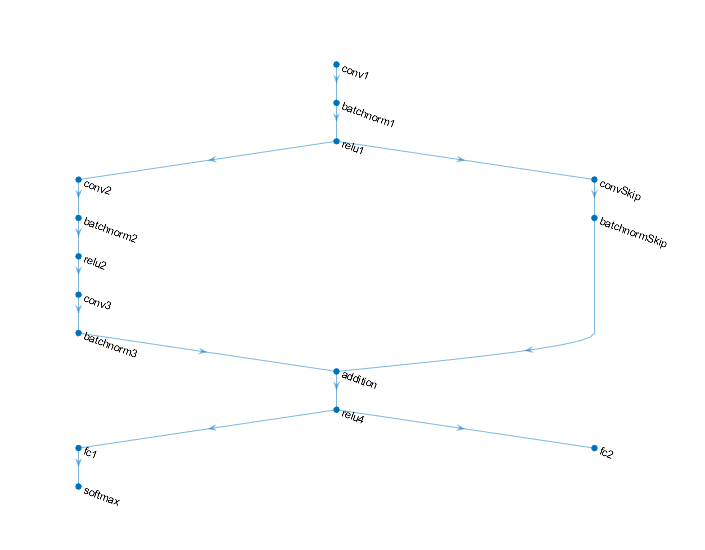## Train Network Using Model Function

This example shows how to create and train a deep learning network by using functions rather than a layer graph or a `dlnetwork`. The advantage of using functions is the flexibility to describe a wide variety of networks. The disadvantage is that you must complete more steps and prepare your data carefully. This example uses images of handwritten digits, with the dual objectives of classifying the digits and determining the angle of each digit from the vertical.

### Import Data

The `digitTrain4DArrayData` function loads the images, their classifications, and their angles from the vertical. Load the data, and find the classification categories of the handwritten digits.

```[XTrain,YTrain,ThetaTrain] = digitTrain4DArrayData; cats = categories(YTrain); numClasses = numel(cats); n = length(ThetaTrain); disp(cats)```
``` '0' '1' '2' '3' '4' '5' '6' '7' '8' '9' ```

View a subset of the images.

```idx = randperm(numel(YTrain),64); I = imtile(XTrain(:,:,:,idx)); figure imshow(I)```View the angles of the same subset of images.

`disp(reshape(ThetaTrain(idx),8,8)')`
``` 9 -18 21 5 -5 21 -31 13 -24 -11 -17 12 -6 -6 39 -38 -8 -15 -14 -42 -28 -44 -23 7 -2 21 11 -38 3 -18 30 44 -34 40 33 -20 12 -36 43 -39 -30 -30 -30 27 38 -15 25 9 14 45 43 -17 36 -11 39 10 -29 -6 -41 -23 43 -33 2 -29 ```

### Create Model Function and Parameters

A model function is the response of the network to data. The easiest way to create a model function is to use built-in toolbox functions. The `model` helper function passes data through three sets of convolution-batch norm-ReLU steps, followed by fully connected steps with 10 categories for digit classification and one category for digit angle. The function has two outputs: a vector of probabilities for classification and a predicted angle.

The code for the `model` helper function is at the end of this example.

The `model` function uses a structure of parameters, where the parameters are the deep learning network weights. To initialize the model for training, you must give values for the parameters. The recommended approach is to set many of the initial parameters to nonzero values. For those parameters, use a single-precision Gaussian distribution with mean 0 and standard deviation 0.01 by calling the `initializeGaussian` helper function, which appears at the end of this example.

To enable automatic differentiation, create the parameter structure as `dlarray` entries.

```parameters.W1 = dlarray(initializeGaussian([5,5,1,16])); parameters.b1 = dlarray(zeros(16,1,'single')); parameters.Offset1 = dlarray(zeros(16,1,'single')); parameters.Scale1 = dlarray(ones(16,1,'single')); parameters.Ws = dlarray(initializeGaussian([1,1,16,32])); parameters.bs = dlarray(zeros(32,1,'single')); parameters.W2 = dlarray(initializeGaussian([3,3,16,32])); parameters.b2 = dlarray(zeros(32,1,'single')); parameters.Offset2 = dlarray(zeros(32,1,'single')); parameters.Scale2 = dlarray(ones(32,1,'single')); parameters.W3 = dlarray(initializeGaussian([3,3,32,32])); parameters.b3 = dlarray(zeros(32,1,'single')); parameters.Offset3 = dlarray(zeros(32,1,'single')); parameters.Scale3 = dlarray(ones(32,1,'single')); parameters.W4 = dlarray(initializeGaussian([10,1568])); parameters.b4 = dlarray(zeros(numClasses,1,'single')); parameters.R1 = dlarray(initializeGaussian([1,1568])); parameters.B5 = dlarray(zeros(1,1,'single'));```

To create an objective function, you must combine the model function outputs into a scalar value. The `modelGradients` helper function computes the classification loss as the cross entropy of classification, and adds 5% of the regression root mean square error to create the objective. In addition, using automatic differentiation, the function also computes the gradient of the objective. The code for the `modelGradients` helper function appears at the end of this example.

### Specify Training Options

To train the network, use stochastic gradient descent with momentum (sgdm). The equations that define the training loop are

`${w}_{n+1}={w}_{n}-\nabla {f}_{n}L+\alpha \left({w}_{n}-{w}_{n-1}\right).$`

Here ${w}_{n}$ is the weight (parameter) vector at iteration $n$, $\nabla {f}_{n}$ is the gradient of the objective function at iteration $n$, $L$ is the learn rate, and $\alpha$ is the momentum term. Initialize the velocity `vel` as empty (`[]`); `vel` represents ${w}_{n}-{w}_{n-1}$.

`velocity = [];`

The `sgdmupdate` function performs the training loop using the default values $L=0.01$ and momentum $\alpha =0.9$. Set the number of training epochs to 20.

`numEpochs = 20;`

Specify a mini-batch size of 100.

`miniBatchSize = 100;`

Specify to plot the training progress.

`plots = "training-progress";`

Train on a GPU if one is available. Using a GPU requires Parallel Computing Toolbox™ and a CUDA® enabled NVIDIA® GPU with compute capability 3.0 or higher. If you do not have a GPU, set `executionEnvironment` to `"cpu"`.

`executionEnvironment = "auto";`

### Train Model

Initialize the training progress plot.

```if plots == "training-progress" figure lineLossTrain = animatedline; xlabel("Iteration") ylabel("Loss") end```

Loop over the data, shuffling the mini-batch data for each iteration and using the custom training loop updates for the parameter structure.

```iteration = 0; start = tic; for epoch = 1:numEpochs p = randperm(n); for i = 1:miniBatchSize:n iteration = iteration + 1; x = XTrain(:,:,:,i:(i+miniBatchSize-1)); X = single(x); Y = YTrain(i:(i+miniBatchSize-1)); T = cast(cats == Y','single'); T2 = ThetaTrain(i:(i+miniBatchSize-1)); T2 = single(T2); dlX = dlarray(X,'SSCB'); if (executionEnvironment == "auto" && canUseGPU) || executionEnvironment == "gpu" dlX = gpuArray(dlX); end [loss, grad] = dlfeval(@(X, T, T2, parameters)modelGradients(X, T, T2, parameters),dlX,T,T2,parameters); [parameters,velocity] = sgdmupdate(parameters,grad,velocity); if plots == "training-progress" D = duration(0,0,toc(start),'Format','hh:mm:ss'); addpoints(lineLossTrain,iteration,double(gather(extractdata(loss)))) title("Epoch: " + epoch + ", Elapsed: " + string(D)) drawnow end end end```### Compute Model Accuracy on Test Data

The `digitTest4DArrayData` function loads test images and their associated categories and angles.

`[XTest,YTest,ThetaTest] = digitTest4DArrayData;`

Use the `digitAngleModel` function to classify the test images based on the trained parameter structure, and compare the classifications with the true values.

```[YPred,ThetaPred] = model(dlarray(XTest,'SSCB'), parameters); [~,I] = max(extractdata(YPred),[],1); C = cats(I); accuracy = 100*(sum(C==YTest)/numel(C))```
```accuracy = 99.5400 ```

The trained model classifies the test digits quite well.

Find the resulting root mean square error of the predicted digit angles compared to the true angles.

```th = extractdata(ThetaPred); th = th(:); anglerms = sqrt(mean((th - ThetaTest).^2))```
```anglerms = single 7.3652 ```

The angle predictions are noisier than the digit predictions.

View 10 random digit angles compared to their true values.

```r = randperm(length(YTest),10); tt = table(th(r),ThetaTest(r),'VariableNames',{'Predicted','True'})```
```tt=10×2 table Predicted True _________ ____ -7.2183 -5 35.014 38 -32.087 -34 12.619 19 -26.397 -13 31.299 30 -9.4692 -9 -29.969 -32 -28.972 -32 24.677 36 ```

### Helper Functions

The following code is for the `model` helper function.

``` function [dlY1,dlY2] = model(dlX,parameters) dlY1 = dlconv(dlX,parameters.W1,parameters.b1,'Padding',2); dlY1 = batchnorm(dlY1,parameters.Offset1,parameters.Scale1); dlY1 = relu(dlY1); dlYbranch = dlconv(dlY1,parameters.Ws,parameters.bs,'Stride',2); dlYbranch = batchnorm(dlYbranch,parameters.Offset2,parameters.Scale2); dlY1 = dlconv(dlY1,parameters.W2,parameters.b2,'Padding',1,'Stride',2); dlY1 = batchnorm(dlY1,parameters.Offset2,parameters.Scale2); dlY1 = relu(dlY1); dlY1 = dlconv(dlY1,parameters.W3,parameters.b3,'Padding',1); dlY1 = batchnorm(dlY1,parameters.Offset3,parameters.Scale3); dlY1 = dlY1 + dlYbranch; dlY1 = relu(dlY1); dlY1 = avgpool(dlY1,2,'Stride',2); dlY2 = fullyconnect(dlY1,parameters.R1,parameters.B5); dlY1 = fullyconnect(dlY1,parameters.W4,parameters.b4); dlY1 = softmax(dlY1); end```

The following code is for the `initializeGaussian` helper function.

```function parameter = initializeGaussian(parameterSize) parameter = randn(parameterSize, 'single') .* 0.01; end```

The following code is for the `modelGradients` helper function.

```function [loss, grad] = modelGradients(dlX,T,T2,parameters) [y,y2] = model(dlX,parameters); loss = crossentropy(y,T) + 0.05*sqrt(mean((y2(:)-T2(:)).^2)); grad = dlgradient(loss,parameters); end```